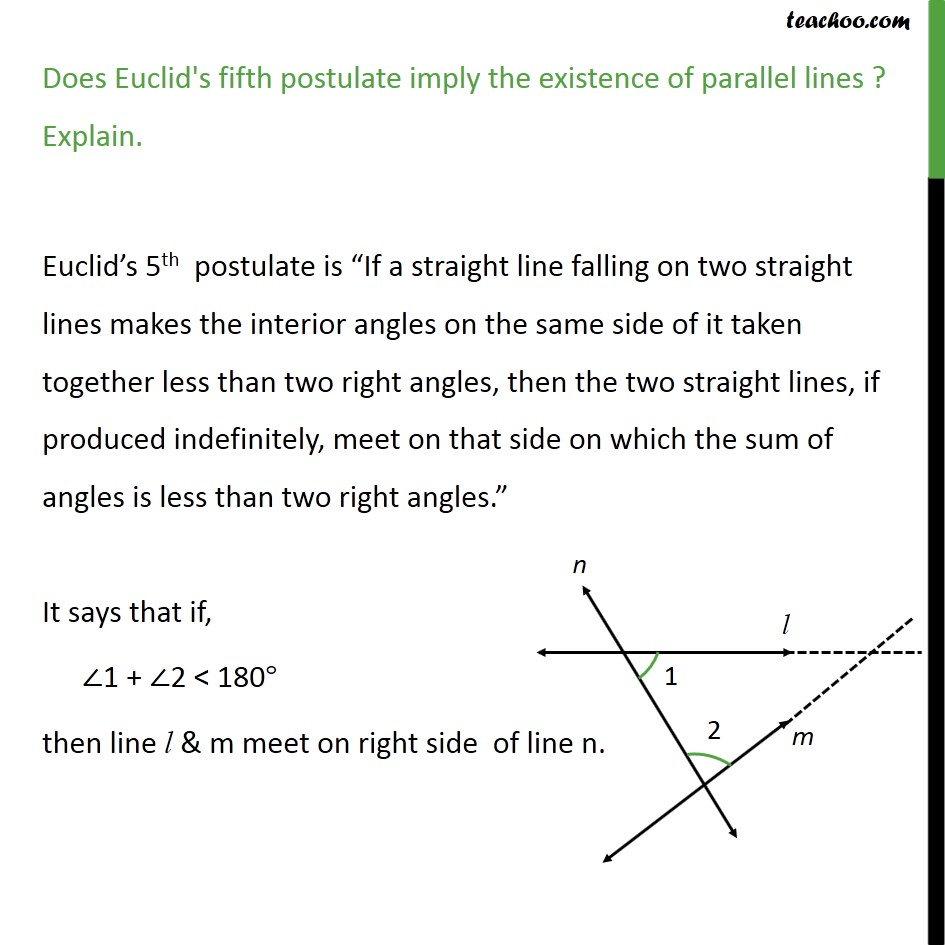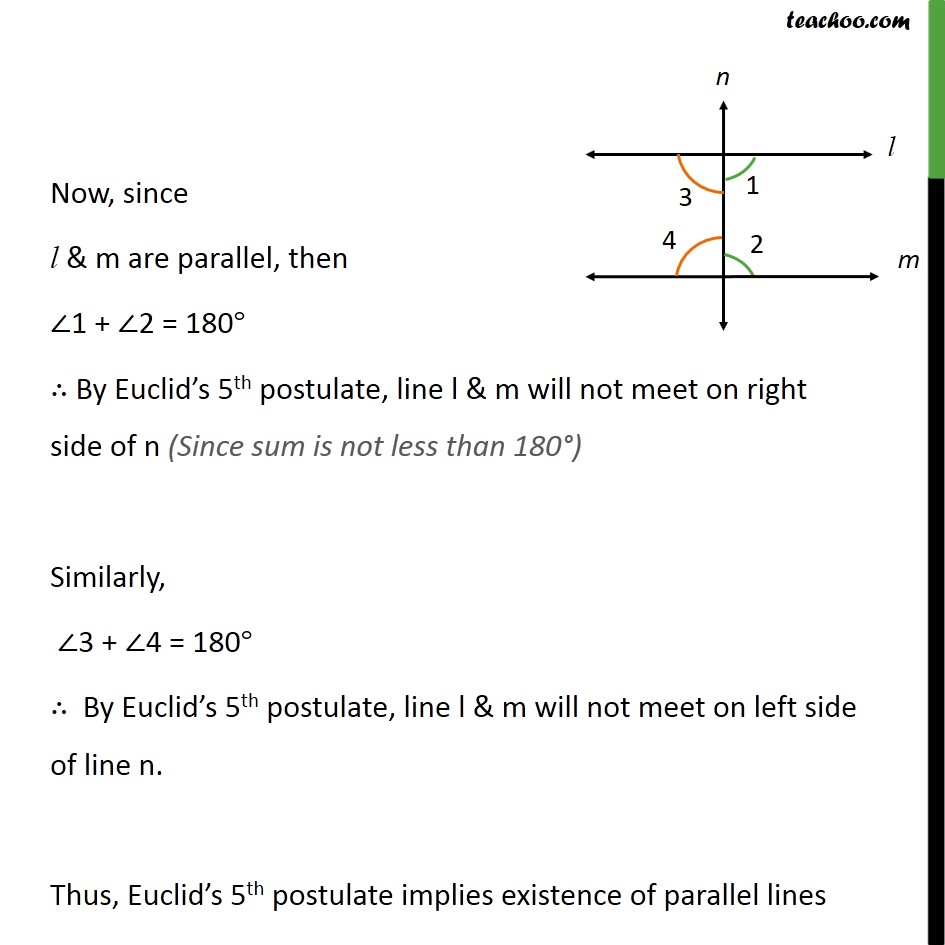Euclid's 5th Postulate

Chapter 5 Class 9 Introduction to Euclid's Geometry
Serial order wiseLearn in your speed, with individual attention - Teachoo Maths 1-on-1 Class

### Transcript

Question 2 Does Euclid s fifth postulate imply the existence of parallel lines? Explain. Postulate 5 : If a straight line falling on two straight lines makes the interior angles on the same side of it taken together less than two right angles, then the two straight lines, if produced indefinitely, meet on that side on which the sum of angles is less than two right angle If a straight line PQ falls on two straight lines AB & CD such that 1 + 2 = 180 & 1 = 90 , 2 = 90 Then lines AB & CD wont meet, So, lines AB & CD are parallel.